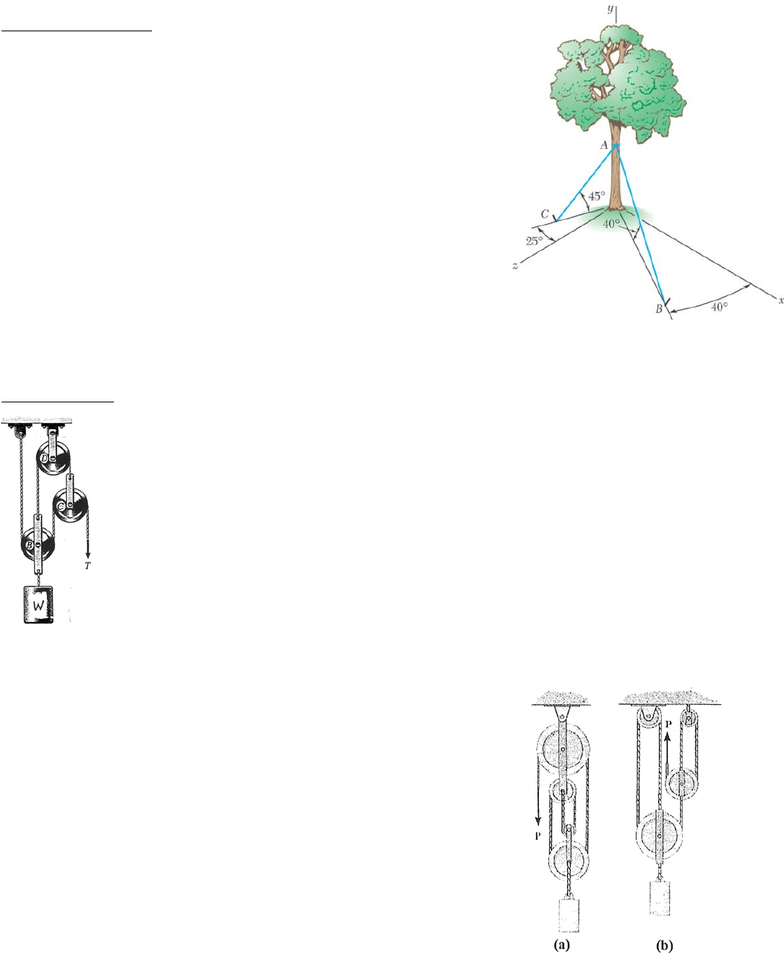# ENGG 202 Lecture Notes - Lecture 1: Unit Vector

194 views3 pagesENGG 202 Tutorial 4 Jan 29/30, 2013
*question is from an old ENGG 205 exam
Review – 3D vectors
1) To stabilize a tree partially uprooted in a storm, cables AB and AC
are attached to the upper trunk of the tree and then are fastened to
steel rods anchored in the ground. Knowing that the tension in cable
AB is 4.2 kN, determine (a) the components of the force exerted by
this cable on the tree and (b) the angles θx, θy, θz for this force.
ANS: F=2.46i-2.70j+2.07k (kN), (b) 54.1o, 130.0o, 60.5o
Solution Strategy: a) to find the components, determine the unit
vector for the direction AB (find position vector from the dimensions
and divide by the magnitude) then multiply the unit vector by the
force magnitude.
b) the components of the unit vector for direction AB found in the
intermediate step in (a) are the direction cosines. Therefore, to find
the angle, take the inverse cosine of each component of the unit vector. Or use the equations from
2D Equilibrium
2*) Given the tension force T = 10 kN, determine the
weight of the block, W, necessary for the pulley-cable
system to be in equilibrium. Neglect the weight of the
pulleys.
ANS: W = 40 kN
Solution Strategy: there are 2 cables in this system, cable 1 over pulleys C
and B and cable 2 over pulley D. each cable has a different tension.
Drawing FBDs of pulley C and pulley B and applying your equations of
equilibrium will allow you to solve for the force in cable 2 in terms of the
cable 1 tension and then to solve for the weight W.
3*) For each case (a) and (b), determine the force P required to
maintain equilibrium. The block weighs 100 N. Which cable-pulley
system is more efficient?
ANS: (a) P = 25 N, (b) P = 11.1 N (more efficient)
Solution Strategy: in case (a) there is only one cable. So drawing
the FBD for the bottom 2 pulleys together (cut through the cable 4
times and include the weight) will allow you to solve for P
For case (b) there are two cables, one that passes over the two pulleys
on the right and one that passes over the two left pulleys. Drawing
the FBDs for the largest pulley will allow you to solve for the force in
one of the cables in terms of the weight, then drawing the FBD for the
next largest pulley will allow you to solve for the force in the other
cable which is P.
Unlock document

This preview shows page 1 of the document.
Unlock all 3 pages and 3 million more documents.